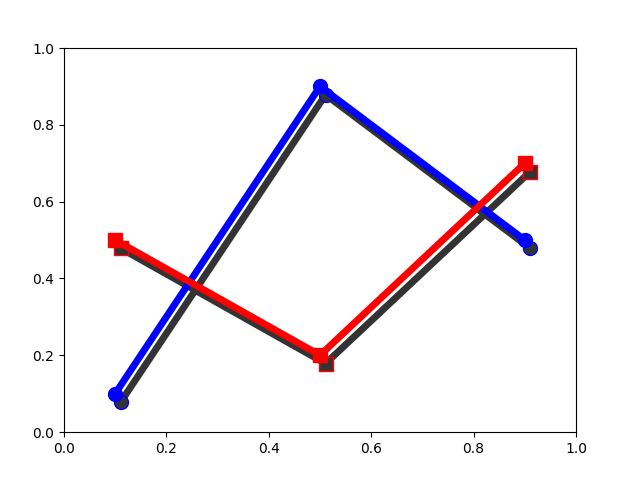# SVG Filter Line¶

Demonstrate SVG filtering effects which might be used with Matplotlib.

Note that the filtering effects are only effective if your SVG renderer support it.Out:

Saving 'svg_filter_line.svg'


import io
import xml.etree.ElementTree as ET

import matplotlib.pyplot as plt
import matplotlib.transforms as mtransforms

fig1 = plt.figure()
ax = fig1.add_axes([0.1, 0.1, 0.8, 0.8])

# draw lines
l1, = ax.plot([0.1, 0.5, 0.9], [0.1, 0.9, 0.5], "bo-",
mec="b", lw=5, ms=10, label="Line 1")
l2, = ax.plot([0.1, 0.5, 0.9], [0.5, 0.2, 0.7], "rs-",
mec="r", lw=5, ms=10, label="Line 2")

for l in [l1, l2]:

# draw shadows with same lines with slight offset and gray colors.

xx = l.get_xdata()
yy = l.get_ydata()

# adjust zorder of the shadow lines so that it is drawn below the
# original lines

# offset transform
ot = mtransforms.offset_copy(l.get_transform(), fig1,
x=4.0, y=-6.0, units='points')

# set the id for a later use

ax.set_xlim(0., 1.)
ax.set_ylim(0., 1.)

# save the figure as a bytes string in the svg format.
f = io.BytesIO()
plt.savefig(f, format="svg")

# filter definition for a gaussian blur
filter_def = """
<defs xmlns='http://www.w3.org/2000/svg'
<feGaussianBlur result='blur' stdDeviation='3'/>
</filter>
</defs>
"""

# read in the saved svg
tree, xmlid = ET.XMLID(f.getvalue())

# insert the filter definition in the svg dom tree.
tree.insert(0, ET.XML(filter_def))

for l in [l1, l2]:
# pick up the svg element with given id